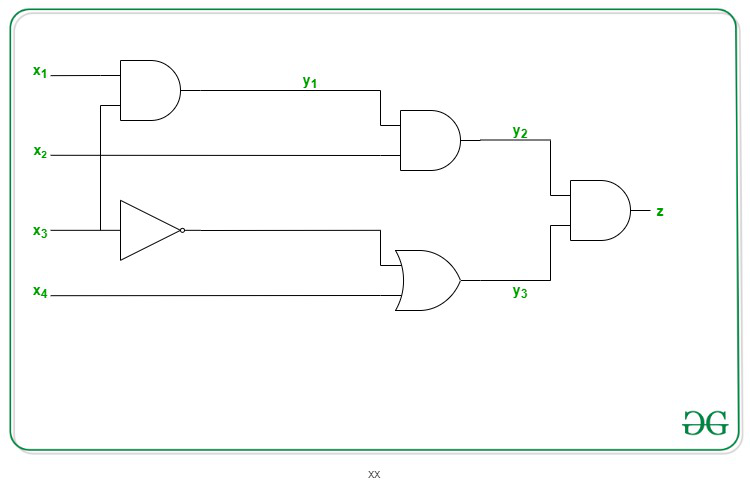# Proof that SAT is NP Complete

• Difficulty Level : Hard
• Last Updated : 14 Oct, 2020

SAT Problem: SAT(Boolean Satisfiability Problem) is the problem of determining if there exists an interpretation that satisfies a given boolean formula. It asks whether the variables of a given boolean formula can be consistently replaced by the values TRUE or FALSE in such a way that the formula evaluates to TRUE. If this is the case, the formula is called satisfiable. On the other hand, if no such assignment exists, the function expressed by the formula is FALSE for all possible variable assignments and the formula is unsatisfiable.

Problem: Given a boolean formula f, the problem is to identify if the formula f has a satisfying assignment or not.

Explanation: An instance of the problem is an input specified to the problem. An instance of the problem is a boolean formula f. Since an NP-complete problem is a problem which is both NP and NP-Hard, the proof or statement that a problem is NP-Complete consists of two parts:

1. The problem itself is in NP class.
2. All other problems in NP class can be polynomial-time reducible to that.
(B is polynomial-time reducible to C is denoted as ≤ PC)

If the 2nd condition is only satisfied then the problem is called NP-Hard.

But it is not possible to reduce every NP problem into another NP problem to show its NP-Completeness all the time i.e., to show a problem is NP-complete then prove that the problem is in NP and any NP-Complete problem is reducible to that i.e. if B is NP-Complete and B ≤ PC For C in NP, then C is NP-Complete. Thus, it can be verified that the SAT Problem is NP-Complete using the following propositions:

SAT is in NP:
It any problem is in NP, then given a ‘certificate’, which is a solution to the problem and an instance of the problem(a boolean formula f) we will be able to check (identify if the solution is correct or not) certificate in polynomial time. This can be done by checking if the given assignment of variables satisfies the boolean formula.

SAT is NP-Hard:
In order to prove that this problem is NP-Hard then reduce a known problem, Circuit-SAT in this case to our problem. The boolean circuit C can be corrected into a boolean formula as:

• For every input wire, add a new variable yi.
• For every output wire, add a new variable Z.
• An equation is prepared for each gate.
• These sets of equations are separated by values and adding Z at the end.

This transformation can be done in linear time. The following propositions now hold:

• If there is a set of input, variable values satisfying the circuit then it can derive an assignment for the formula f that satisfies the formula. This can be simulated by computing the output of every gate in the circuit.
• If there is a satisfying assignment for the formula f, this can satisfy the boolean circuit after the removal of the newly added variables.

For Example: If below is the circuit then:Therefore, the SAT Problem is NP-Complete.

My Personal Notes arrow_drop_up